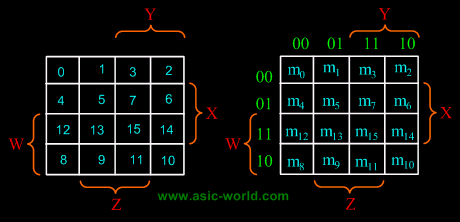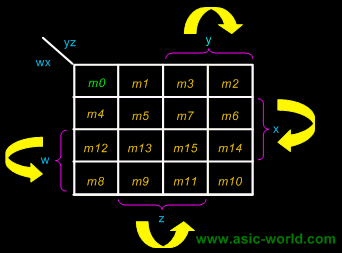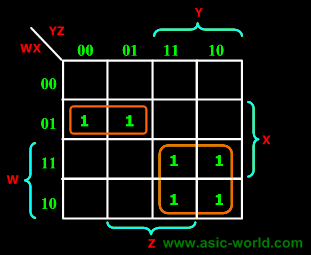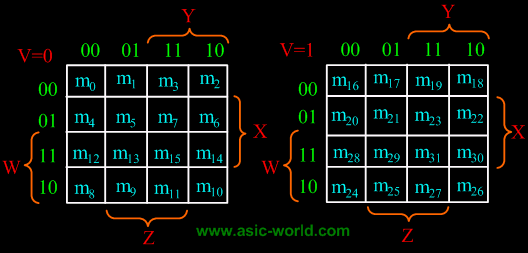# Trung tâm đào tạo thiết kế vi mạch Semicon

• Đăng ký
 Name: * Tên đăng nhập: * Email: * Mật khẩu: * Verify Password: * Fields marked with an asterisk (*) are required.## Simplification Of Boolean Functions Part-III4-Variable K-Map

There are 16 cells in a 4-variable (W, X, Y, Z); K-map as shown in the figure below.There are 2 wrap-around: a horizontal wrap-around and a vertical wrap-around. Every cell thus has 4 neighbours. For example, the cell corresponding to minterm m0 has neighbours m1, m2, m4 and m8Example

F(W,X,Y,Z) = (1,5,12,13)F = WY'Z + W'Y'Z
Example

F(W,X,Y,Z) = (4, 5, 10, 11, 14, 15)
F = W'XY' + WY5-Variable K-Map

There are 32 cells in a 5-variable (V, W, X, Y, Z); K-map as shown in the figure below.
Inverse Function

• The 0's on a K-map indicate when the function is 0.
• We can minimize the inverse function by grouping the 0's (and any suitable don't cares) instead of the 1's.
• This technique leads to an expression which is not logically equivalent to that obtained by grouping the 1's (i.e., the inverse of X != X').
• Minimizing for the inverse function may be particularly advantageous if there are many more 0's than 1's on the map.
• We can also apply De Morgan's theorem to obtain a product-of-sum expression.
•   Bạn Có Đam Mê Với Vi Mạch hay Nhúng      -     Bạn Muốn Trau Dồi Thêm Kĩ Năng

# Hãy Để Chúng Tôi Hỗ Trợ Cho Bạn. SEMICONLần cập nhật cuối ( Thứ ba, 29 Tháng 3 2022 00:13 )